# E-PolyLearning

 16. If xD = overhead product molal composition and RD = reflux ratio, then slope and intercept of the operating line for rectifying section are respectively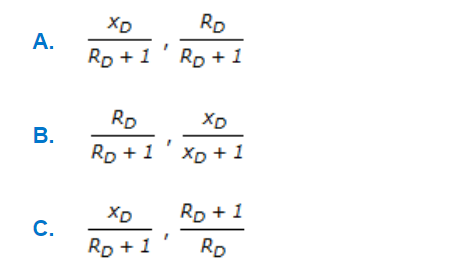a. A b. B c. C d. None of these

 17. Below is given an equilibrium moisture curve of a substance. State which regions represent free moisture and unbound moisture respectively.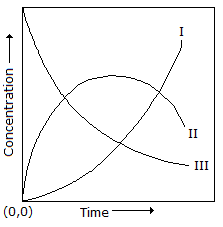a. II, III b. II, IV c. IV, III d. IV, II
 18. The following expression is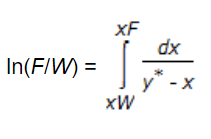a. differential distillation equation b. for NtoG in absorption for dilute mixture c. slope of q-line in McCabe-Thiele method d. for relative volatility
 19. Which of the following is the correct ternery diagram for liquid-liquid extraction system comprising of one pair partly soluble (e.g. water-benzene-acetic acid in which A-diluent, B - solvent and C - solute)?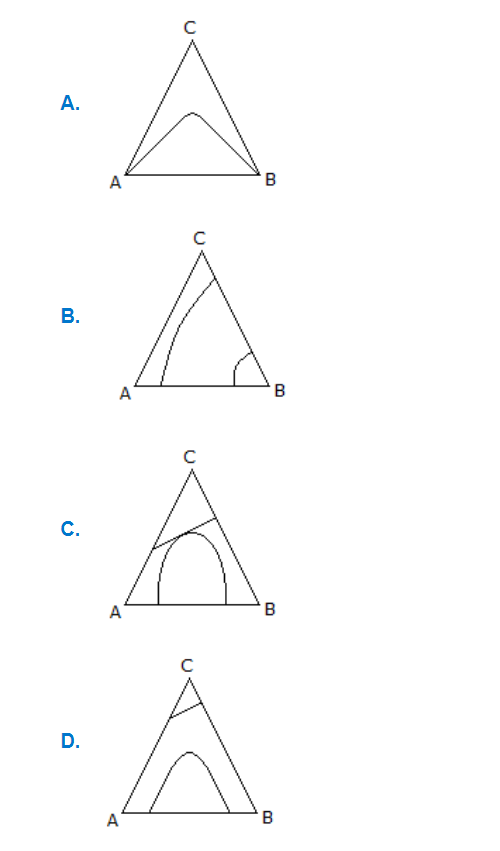a. A b. B c. C d. D
 20. The velocity, concentration and temperature boundary, for the boundary layer development on a flat plate during connective mass transfer will be same, if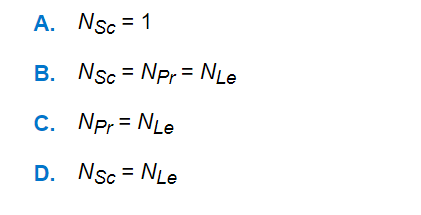a. A b. B c. C d. D